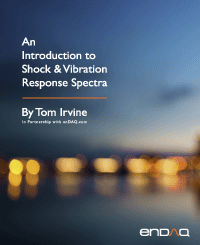Sine Sweep Testing
By Tom Irvine
SECTION 1

# Sine Sweep Introduction

Structures and components may be mounted on shaker tables, similar to those in Figure 4.9 and Figure 4.10, and subjected to sine sweep base excitation. The essence of a sine sweep test is that the base excitation input consists of a single frequency at any given time. The frequency itself, however, is varied with time. The sine sweep test may begin at a low frequency and then sweep to a high frequency, or vice-versa. Some specifications require several cycles, where one cycle is defined as from low to high frequency and then from high back to low frequency.

The specification might require either a linear or a logarithmic sweep rate. The sweep will spend greater time at the lower frequency end if the sweep is logarithmic. The example in Figure 13.1 had a logarithmic sweep rate and a constant amplitude. Nevertheless, the specification might require that the amplitude vary with frequency.

Some potential test objectives are:

• Identify natural frequencies and amplification factors or damping ratios
• Perform sine sweep before and after random vibration test to determine if any parts loosened, etc.
• Check for linearity of stiffness and damping by varying the input amplitude
• Workmanship screen for defective parts and solder joints
• Represent an actual environment such as a rocket motor oscillation
• NASA/GSFC typically uses sine sweep vibration for spacecraft testing
SECTION 2

## Sine Sweep Time Domain Equations

Linear Sweep Rate

The normalized amplitude function for a linear sweep is

The test duration is T. The starting and ending frequencies are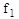and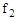, respectively.

Logarithmic Sweep Rate

The normalized amplitude function for a logarithmic sweep is

The sweep rate in octaves per time is R. Recall the octave formula Section 5.6. A typical sweep rate is one octave/minute for sine sweep tests intended for natural frequency identification.

Sweep Type Comparison

A comparison of the frequency time variation for two sweep rate types is shown in Figure 1.2. The sweeps were performed over the frequency domain from 20 to 2000 Hz over a 400 second duration. This is equivalent to a rate of one octave/minute for the logarithmic sweep.

SECTION 3
Sine Sweep Specification Example

A vendor has a product that must withstand sinusoidal vibration with an amplitude of 12 G, over the frequency domain from 10 Hz to 2000 Hz, with a logarithmic sweep rate.

The shaker table has a displacement limit of 1.0 inch peak-to-peak, or 0.5 inch zero-to-peak. Recall that the displacement limit is a constraint at low frequencies. How should the test be specified? The answer is to use a specification with two amplitude segments as shown in Figure 1.3. The first segment is a constant displacement ramp, 0.5 inch zero-to-peak. The second segment is a constant acceleration plateau at 12 G.

The cross-over frequency is calculated from the square root of the ratio of the peak acceleration to the peak displacement. The respective peaks should be in zero-to-peak format.

The cross-over frequency for the specification in Figure 11.2 is

This is the frequency at which the 12 G acceleration corresponds to a 0.5 in zero-to-peak displacement. It is the knee frequency in Figure 1.3.

SECTION 4
Sine Sweep Input & Response Example, SDOF System

The SDOF system in Figure 12.12 has a natural frequency of 40 Hz and amplification factor Q=10. It is subjected to a base input of 1 G from 10 to 80 Hz, with a logarithmic rate of 1 octave/min. The input and response time histories are shown in Figure 1.4 and Figure 13.15. The peak response is 10 times the peak input acceleration. This corresponds to Q=10, but this is the special of a single-degree-of-freedom excited into resonance. A better method for damping identification is the half-power bandwidth method, which is particularly needed for multi-degree-of-freedom and continuous systems due to modal gain factors.

A transmissibility function can be made relating the response to the input in the frequency domain. This is done by tracking the frequency in terms of the zero-crossing rate. The result is shown in Figure 1.6 along with the half-power bandwidth calculation. Each of the points in Figure 13.6 are approximations constrained by the resolution and plot cursor limitations. The points at 37.85 and 41.91 Hz are each half-power points, 3 dB less than the resonant peak. The amplification factor is the frequency difference between the half-power points divided by the center frequency.

Recall that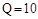corresponds to 5% damping.

SECTION 5
Sine Sweep Input & Response Example, Continuous Beam
 Material Aluminum Length 24 inch Cross-section Circular, 1 inch diameter Area Moment of Inertia 0.04909 in^4 Elastic Modulus 1.0e+07 lbf/in^2 Mass/Length 0.0785 lbm/in (2.035e-04 lbf sec^2/in^2) Amplification Factor Q=10 for all modes

Consider the cantilever beam in Figure 1.7 with the properties in the previous table.

The fundamental frequency of the cantilever beam using the formula in Table 8.3.

The first four natural frequencies and their associated parameters are shown in the following table.

Table 5.2. Cantilever Beam Modal Parameters
Mode Natural Frequency (Hz) Participation Factor Modal Mass Ratio
1 47.7 2.99E-03 0.646
2 299 9.20E-04 0.198
3 837 3.16E-04 0.068
4 1641 1.62E-04 0.035

The cantilever beam is subjected to a 1 G sine sweep from 20 to 2000 Hz at a logarithmic rate of one octave/minute. The acceleration response is shown in Figure 1.8, as calculated using Reference . The corresponding transmissibility function is shown in Figure 1.9.

Again, each of the modes had an amplification factor of 10, but each of the four peaks in Figure 1.9 has an amplitude other than 10. The peaks depend on both the amplification factor and the mode shape parameters. Nevertheless, the half-power bandwidth method can be used to extract the proper amplification values as show for the first and second modes in the following equations.

The amplification factor for the first bending mode is

The amplification factor for the second bending mode is

The half-power bandwidth calculation could be repeated for the third and fourth modes.

SECTION 6
Random Excitation Sources

The liftoff acoustic event occurs during the first two seconds. The vibration then tapers down to a lower lever during the subsonic portion of flight. The envelope then increases as the vehicle accelerates through the transonic velocity and encounters its maximum dynamic pressure condition in the 10 to 20 second segment. The level then gradually decreases throughout the remainder of the first stage burn. Some transient events occur beginning at 60 seconds due to the attitude control system and other sources.

Consider the following vibration sources:

• Turbulent airflow passing over an aircraft wing
• Oncoming turbulent wind against a building
• Rocket vehicle liftoff acoustics
• Earthquake excitation of a building

One common characteristic of these examples is that the motion varies randomly with time. Thus, the amplitude cannot be expressed in terms of a "deterministic" mathematical function.

Dave Steinberg wrote :

The most obvious characteristic of random vibration is that it is nonperiodic. A knowledge of the past history of random motion is adequate to predict the probability of occurrence of various acceleration and displacement magnitudes, but it is not sufficient to predict the precise magnitude at a specific instant.

Random vibration analysis is thus a matter of probability and statistics. The parameters include: mean, standard deviation, histogram, power spectral density, etc. Stationary random vibration is where the key statistical parameters remain constant over time. The goal of most shaker table vibration tests is to apply a stationary base input to the test unit. This would be the case where the specification was a power spectral density. But real-world vibration tends to be nonstationary as show in Figure 1.10.

SECTION 7
Statistical Parameters

The mean value μ of the time history is calculated as

whereis the instantaneous amplitude and n is the total number of points.

The varianceof the time history is

The root-mean-square value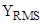of the time history is

A normal distribution is symmetric about its mean value, but some time histories may have skewed histograms. The skewnessis calculated as

Kurtosis is a measure of the "tailedness" of the histogram. The kurtosis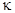is calculated as

Sinusoidal time histories have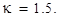Broadband random, stationary, Gaussian time histories have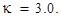Time histories with higher kurtosis values usually have higher sigma peaks than those expected from a Gaussian distribution.

The crest factor is the ratio of the absolute peak divided by the RMS.

The denominator on the right-hand side may be replaced by the standard deviation for the case of zero mean. The crest factor for pure sine vibration is always √2. The crest factor for random vibration is typically assumed to be 3, but higher crest factors can occur even for closed-loop stationary broadband vibration test performed on shaker tables .

Now consider a single-degree-of-freedom system subjected to a stationary Gaussian broadband random, applied force or base excitation. The expected crest factor Ce per Reference  is

Note that equation (1.15) can be extended to a multi-degree-of-freedom response by replacing the natural frequency with the positive-slope zero-crossing frequency.

SECTION 8
Random Vibration Histograms

Again, the histogram divides the time history amplitude points into bins and then counts the points in each bin. The fundamental histogram for random vibration is the Gaussian or Normal distribution, also known as a bell curve. This function represents the distribution of the instantaneous points for a stationary random vibration environment, which may either be broadband or narrowband. White noise is a special case of broadband vibration which is present later in this document. The Gaussian histogram can be normalized as probability density function such that the total area under the curve is equal to one. These functions are useful in vibration analysis for estimating peak responses and fatigue damage.

Again, the mean value is μ and the standard deviation is σ. The mean value in random vibration is usually zero, either because the vibrating object has zero net displacement or because the accelerometer signal has been AC coupled.

The Gaussian distribution equation is

The probability that the variable will be between any two given limits is obtained by integrating the area under the curve, which requires the gamma function. Probability examples are shown in the following tables.

Table 8.1. Gaussian Distribution, Probability of Occurrence Between Limits
Statement Probability Ratio Percent
-σ < x < +σ 0.6827 68.27%
-2σ < x < +2σ 0.9545 95.45%
-3σ < x < +3σ 0.9973 99.73%
Table 8.2. Gaussian Distribution, Probability of Occurrence Outside Limits
Statement Probability Ratio Percent
| x | > σ 0.3173 31.73%
| x | > 2σ 0.0455 4.55%
| x | > 3σ 0.0027 0.27%

The Rayleigh distribution is a continuous probability distribution for positive-valued random variable with the equation

The X-axis represents a scale factor times the standard deviation, as denoted by A which is used to denote the absolute value of x.

The area under the curve from zero to a given A value represents probability of occurrence. The total area under the curve is one. The probability of exceeding the A value is one minus the area as shown in 13.5. The probability that a given peak will be within zero and 1σ is 39.35%. The probability of exceeding 1σ is 60.65%.

An SDOF response time history can be reduced to a subset of points representing local peaks. A peak occurs at a point where the initial slope has the opposite polarity of the ending slope. A peak can be negative or positive regardless of whether it is a local minimum or maximum. The peak distribution will tend to be a Rayleigh distribution for random excitation, whether by applied force or base input. Characterizing the distribution is important for fatigue analysis.

Table 8.3. Rayleigh Distribution, Probability of Occurrence Outside Limits
ʎ Prob [ A > ʎσ ]
0.5 88.25 %
1.0 60.65 %
1.5 32.47 %
2.0 13.53 %
2.5 4.39 %
3.0 1.11 %
3.5 0.22 %
4.0 0.034 %

Thus, 1.11 % of the peaks will be above 3σ for a signal whose peaks follow the Rayleigh distribution.

SECTION 9
White & Pink Noise

White light passed through a prism produces a spectrum of colors. White noise is a type of analogous random vibration. White noise and pink noise are two special cases of random vibration.

White noise is a random signal which has a constant power spectrum for a constant frequency bandwidth. It is thus analogous to white light, which is composed of a continuous spectrum of colors. Static noise over a non-operating TV or radio station channel tends to be white noise. In addition, Commercial white noise generators are designed to produce soothing random noise which masks household noise as a sleep aid.

Pink noise is a random signal which has a constant power spectrum for each octave band. This noise is called pink because the low frequency or “red” end of the spectrum is emphasized. Pink noise is used in acoustics to measure the frequency response of an audio system in a particular room. It can thus be used to calibrate an analog graphic equalizer. Waterfalls and oceans waves may generate pink-like noise.

SECTION 10
White Noise Input & Response Example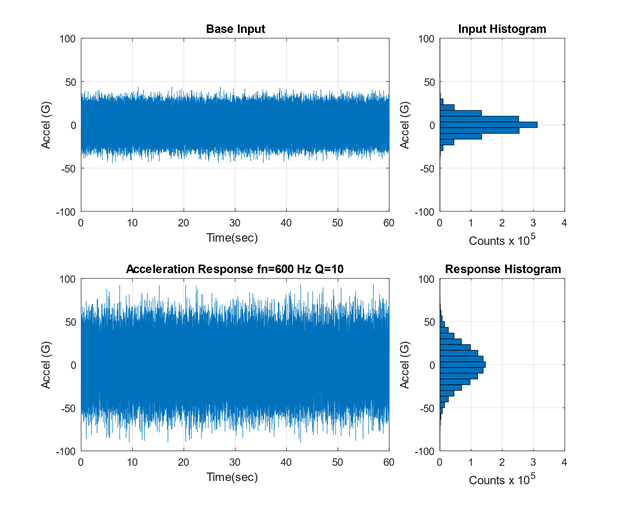Figure 10.1. White Noise Base Input Time History and SDOF Response with Histograms

Stationary white noise base input excitation is applied to the SDOF model in Figure 12.12. The system has a natural frequency of 600 Hz and an amplification factor of Q equal to 10, or 5% damping. The input time history is shown in Figure 1.14, with a Gaussian distribution and a standard deviation of 10 G. The response is calculated using the Smallwood ramp invariant digital recursive filtering relationship . The response likewise has a Gaussian distribution.Figure 10.2. SDOF Response to White Noise Base Input, Close-up View
Table 10.1. SDOF Response to White Noise Statistics
Parameter Value Parameter Value
Mean 0 G Kurtosis 3.0
Std Dev 21.8 G Crest Factor 4.28
RMS 21.8 G Maximum 93.3 G
Skewness 0 Minimum -90.6 G

A brief segment from the input and response are given in Figure 1.15. The white noise input is broadband random. The response tends to vibrate at 600 Hz natural frequency, but the amplitude envelope varies randomly with time. The response is narrowband random. The response statistics for the entire duration are given in Table 1.6.

The response mean is zero. The RMS and standard deviation values are thus equal per equation (17) and have a value of 21.8 G. The highest input absolute peak is 93.3 G. The ratio of the peak relative to the standard deviation is called the “crest factor,” which is 4.28 in this example. This value can also be represented as 4.28σ. A common assumption is that the crest factor is 3σ, which is three times the standard deviation. But 4σ and 5σ peaks are common in stationary random vibration.Figure 10.3. SDOF Response to White Noise, Absolute Peak Values with Histogram

The histogram of the absolute local peaks has a Rayleigh distribution. The expected crest factor for the sample problem is

The crest factor for sample problem was 4.28, which is 10% lower than the expected value.

SECTION 11
Crest Factor Study

As a numerical experiment, the white noise synthesis and response calculation steps for the previous example were repeated 20,000 times. A crest factor was calculated for each trial. A histogram of the crest factor is shown in Figure 13.17. The histogram has a shape somewhat similar to a Rayleigh distribution, but further investigation is needed to properly characterize it. The crest factors ranged from 4.14 to 6.62, with a mean of 4.76. This experimental mean value was 1.1% higher than the theoretical 4.71 value. Again, stationary random vibration time history with Gaussian distributions typically have peaks ranging from 4σ to 5σ, but higher crest values may also occur. This is true both for broadband inputs and narrowband responses. Designing structures and components for 3σ vibration loads is thus insufficient, even though this is a common rule-of-thumb.

SECTION 12
Non-Gaussian Random Vibration

Stationary Gaussian random time histories can be generated in closed-loop shaker table tests. But time histories measured in the field usually depart from the Gaussian ideal. A theoretical example is shown in Figure 1.18. Nevertheless, the response of an SDOF system to a non-Gaussian random input will have some tendency to be Gaussian due to the “central limit theorem.”

The central limit theorem in probability theory establishes that, in some situations, when independent random variables are added, their properly normalized sum tends toward a normal distribution even if the original variables themselves are not normally distributed.

The base input has standard deviation=1.0, kurtosis=9.3 and crest factor=11.1. The response statistics are shown in the following table.

Table 12.1. SDOF Response to Non-Gaussian Time History
Parameter Value Parameter Value
Mean 0 G   Kurtosis 3.5
Std Dev 2.12 G   Crest Factor 6.9
RMS 2.12 G   Maximum 12.7 G
Skewness 0   Minimum -14.6 G
SECTION 13
HALT/HASS Testing with Non-Gaussian Random Vibration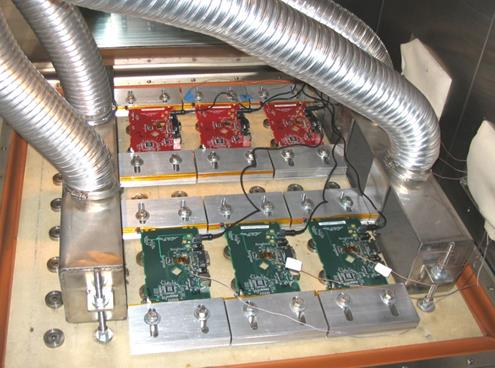Figure 13.1. HALT/HASS Testing of Circuit Boards, Combined Thermal Cycling and Vibration (https://www.dfrsolutions.com/hubfs/Resources/sherlock/How-Sherlock-Can-Help-You-Now-and-Future.pdf)

Highly Accelerated Life Testing (HALT) is a process for ruggedization of preproduction products. Highly Accelerated Stress Screening (HASS) is the production screen for products once they have been characterized in HALT, typically performed on 100% of units per lot. The random vibration environment is nonstationary, non-Gaussian vibration with high kurtosis. The overall GRMS of the vibration is controlled, but the spectral content is uncontrolled. The thermal cycling and vibration may be applied separately or simultaneously depending on the test phase. Note that HALT/HASS is the offspring of the classical Environmental Stress Screening (ESS) process.

The underside of the chamber is connected to hammer impactors which create a repetitive shock time history.

A time history was synthesized to represent a typical HALT chamber vibration environment, as shown in Figure 1.21.

The kurtosis is 8.9 and the crest factor is 9.3. The time history appears stationary, but the close-up view in Figure 13.22 reveals that it is composed of a series of transient pulses.

The vibration is a series of repetitive shock pulses due to the hammer strikes underneath the table.